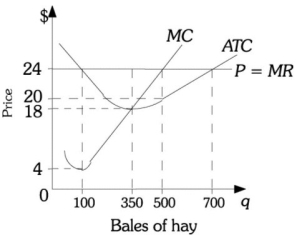# [Solved] Refer to the Information Provided in Figure 8

Question 288
Multiple Choice

## Refer to the information provided in Figure 8.9 below to answer the question(s) that follow.Figure 8.9-Refer to Figure 8.9. If this farmer produces the profit-maximizing level of hay when the market price is \$18 per bale, her total cost would be

A) \$1,200.
B) \$2,800.
C) \$5,600.
D) \$6,300.

10+ million students use Quizplus to study and prepare for their homework, quizzes and exams through 20m+ questions in 300k quizzes.

Explore our library and get Economics Homework Help with various study sets and a huge amount of quizzes and questions

3.5K

Study sets

66.5K

Quizzes

5.9M

Questions

Upload material to get free accessInvite a friend and get free accessSubscribe and get an instant access